### Captures d’écran iPhone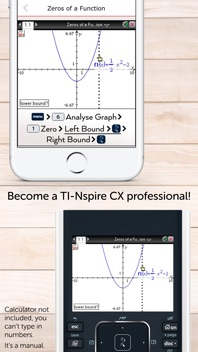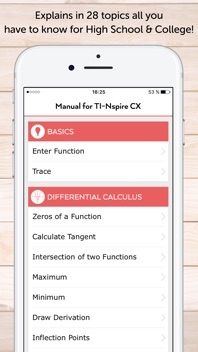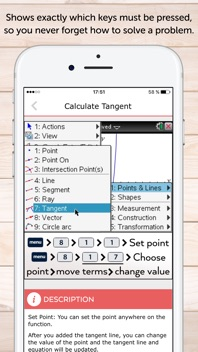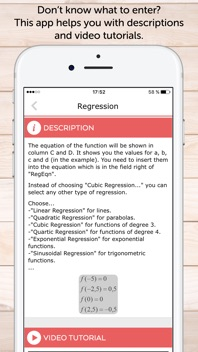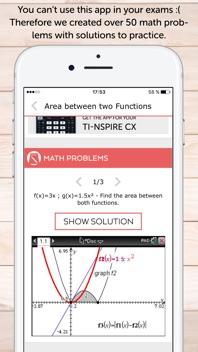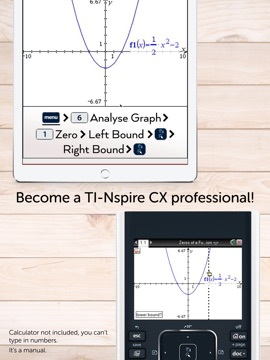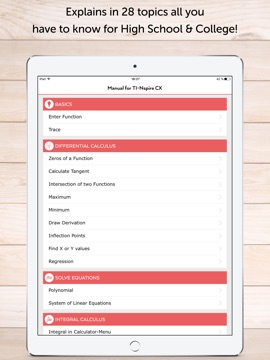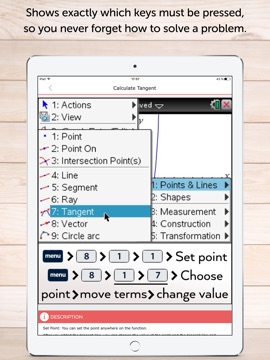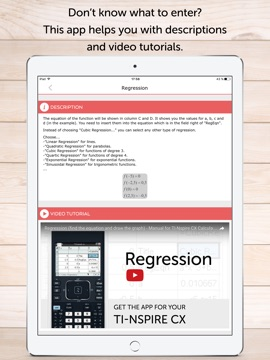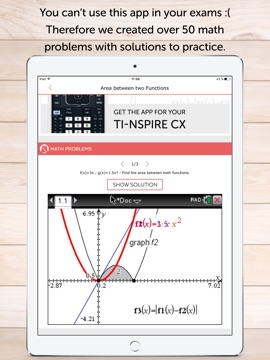### Description

How this app makes math so much easier:
• This manual shows all important functions of the new graphing calculator TI-Nspire CX (and CAS), which are very useful for high school and college. Actual calculator not included!
• The app shows the exact key press sequence for 28 topics of Differential Calculus, Solve Equations, Integral Calculus, Matrices and CAS Functions!
• You can swipe through screenshots of the original graphing calculator which show every step you have to do (220 screenshots in total)!
• Or you can watch short video tutorials to see what you have to do.
• Additionally there are helpful descriptions in the app which give some hints and further knowledge.
• More than 50 math problems with solutions to practice your skills!
Not yet convinced? Here's how you would benefit from this app:
• Every topic shows a detailed instruction through screenshots of the original graphing calculator TI-Nspire CX which show how to solve the math problem. You only have to swipe to get the next screenshot which looks similar with your calculator display!
• To ”become warm” you have the possibility to insert a sample function in your calculator which is used in the app. The app shows an example for every topic. After that, press the first key of the respective key press sequence on the graphing calculator. Then swipe once again to get the next screenshot. It will look like the display of your calculator. The app contains for each step a picture, so it's easier to solve your math problems and become a TI-Nspire CX professional.
• The app is also useful for preparing your examination, because you often don't remember all instructions of the calculator.
• Your teacher will be grateful as well, because he doesn't have to walk around the classroom until everyone's calculator shows the right solution. You only have to take a look at the app and know how to do!
• The app contains the following topics:
BASICS
• Enter Function
• Trace
DIFFERENTIAL CALCULUS
• Zeros of a Function
• Calculate Tangent
• Intersection of two Functions
• Maxima
• Minima
• Draw Derivation
• Inflection Points
• Find X or Y values
• Regression
SOLVE EQUATIONS
• Polynomial
• System of Linear Equations
INTEGRAL CALCULUS
• Find Area with Absolute Value
• Area between two Functions
MATRICES
• Save Matrix
• Put into Row Echelon Form
• Solve Matrix
• Transpose of a Matrix
• Identity Matrix
• Inversion of a Matrix
CAS FUNCTIONS
• Derivative
• Integration
• Expand an Expression
• Solve Equations exactly
• Find Tangent Line
MATH PROBLEMS
• Practice with over 50 math problems and solutions
• You can practice math problems of a single topic (e.g. Zeros of a Function) or random math problems of Differential Calculus for example.
• As an alternative the app shows you mixed math problems of all topics.
We also have apps for the calculators: TI-Nspire CX (and CAS), TI-84 Plus, TI-84 Plus CE, CASIO fx-9860GlI.
CALCULATOR NOT INCLUDED. THE APP IS A MANUAL.

### Nouveautés de la version 1.3.0

+ Updates to all TI-Nspire CX topics
+ Better descriptions
+ Improved User Interface
+ iPhone X support
+ Fixed bugs

### Vendeur

#### Nom

Graphing Calculator Apps UG (haftungsbeschrankt)

### Informations

1.3.0

36,1 MB

Non

Oui

#### Classement

Classification : 4+

#### Compatibilité

iOS 9.0 ou plus récent

anglais

1 note

Pas d'entrée